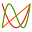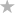#### File Exchange > Graphing >    Chebyshev Polynomial FitAuthor:
OriginLab Technical Support
6/1/2018
Last Update:
6/24/2018
53
Total Ratings:
0
File Size:
148 KB
Average Rating:File Name:
chebyfit.opx
File Version:
1.00
Minimum Versions:
Free
Summary:

Determine the coefficients of Chebyshev polynomials of the first kind.

Description:

Purpose
This app can be used to fit data to Chebyshev polynomial series of the first kind.

$$f(x)=A_{0}\times T_{0}(\bar{x})+A_{1}\times T_{1}(\bar{x})+...+A_{n}\times T_{n}(\bar{x})$$

Each polynomial is represented with normalized argument $$\bar{x}$$ by the recurrence relation

$$T_{0}(\bar{x})=1$$

$$T_{1}(\bar{x})=\bar{x}$$

$$T_{n+1}(\bar{x})=2xT_{n}(\bar{x})-T_{n-1}(\bar{x})$$

Installation
Download the file, then drag-and-drop onto the Origin workspace. An icon will appear in the Apps gallery window.

Operation

1. Activate a worksheet with XY/Y Error columns, or a graph page with data plots.
2. Click the icon in the Apps Gallery window to open the dialog.
3. Select XY columns or data plots as input.
4. Select another column as Y Error (optional). Then you can choose No Weighting, Direct Weighting or Instrumental as how you want to treat errors as weight.
5. Choose polynomial order.
6. Click Preview or check Auto Preview to view fitted curve.
7. Specify quantities and plots to output.
8. Click OK.